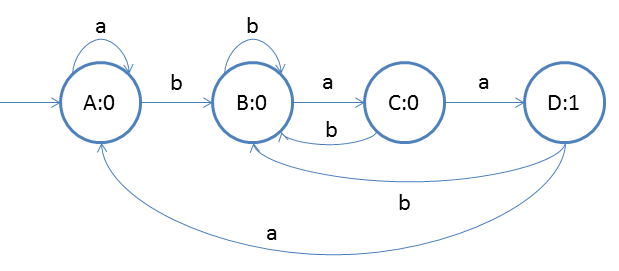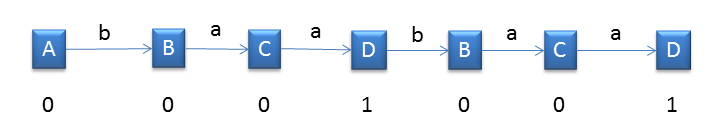Design moore machine for counting baa's in a given string

Firstly we will design a DFA for accpeting baa's then we will write output inside states.Now we will assign the output inside states so that we can count baa's. For accepting state D we will assign '1' and
for other states we will assign '0'.So as you see the ouput inside the states. We will understand the concept more clearly using the below example
Example
We have taken string 'baabaa'. So as you can see from the below diagram that we will get '1' twice.That means there are two 'baa'. That is what we wanted to find.
Number of baa's = Number of '1'
Parsing of string will start from left to right